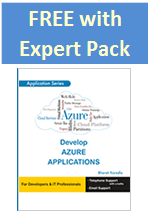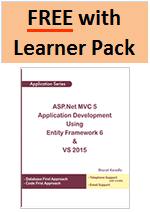# Title: Data Science for ERP Software Developers## Pay only for 2

The objective of this book is to teach the Data Science for ERP Software Developers using python, tensorflow and keras.

### Chapter 1: Introduction to Data science

1. Why this book ?
2. What is Data Science? Getting value out of data
3. Skills necessary for a Data Scientist? Why Python for Data Science?
4. Why Python?
5. Case Study: Soccer Data Analysis
6. Steps in Data Science process
7. Importance of defining the well stated data science problem
8. Steps in data science in detail
9. Acquiring data
10. Exploring Data
11. Pre-Processing Data
12. Scaling and Transformation
13. Dimensionality reduction
14. Data manipulation
15. Analyze Data
16. Classification
17. Regression
18. Clustering
19. Association analysis
20. Reporting Insights
21. Turning Insights into Action

### Chapter 2: Python Programming for Data science

1. Install Python on Windows using Anaconda Python Distribution
2. Why Jupyter Notebook?
3. Python Variables
4. Python Loops
5. for Loop
6. While Loop
7. Python conditions
8. Relational Operators
9. Logical Operators
10. Data Structures - lists
11. Indexing Lists
12. Iterating over a list
13. Modifying Items in Lists
14. Appending to a list
15. Removing from a list
16. Merging two lists using Extend method
17. Iterate over Multiple Lists Simultaneously
18. Applying Functions To List Items
19. List slicing
20. Constructing a List with List Items
21. String functions
22. Concatenation, Strip, Split, Slicing
23. Find a substring
24. String Formatting
25. Tuples in Python
26. Creating a tuple
27. Accessing Values in Tuples
28. Dictionaries
29. Accessing Dictionary Elements with Keys
30. Using Functions to Access Elements
31. Modifying Dictionaries
32. Remove items from a Dictionary
33. Numpy Library
34. Introduction to Arrays
35. Create a rank one ndarray
36. Two-dimensional arrays
37. Create a rank two numpy array
38. Summing the Values in an Array
39. Indexing and Slice indexing
40. Using combinations of integer indexing and slice indexing to create different shaped matrices
41. Numpy: ndarray boolean indexing
42. Numpy: ndarray Datatypes and Operations
43. Arithmetic Array Operations
44. Numpy Statistical Operations
45. Matrix Computations

### Chapter 3: Pandas -Data Analysis Library

1. Introduction to Pandas Library
2. Pandas Data Structures
3. Pandas Series
4. Pandas DataFrame
5. Differences between ndarrays and Series Objects
6. Indexing and Slicing Series
7. Integer-location based indexing Example
8. Series Initialized with Dictionaries
9. DataFrames
10. Example 1:- Create a Dataframe from a Dictionary of equal length lists with columns attribute
11. Example 2:- Create a dataframe from a dictionary of equal length lists with index attribute
12. Example 3 :- Create DataFrame from dictionary of Pandas Series
13. Example 4:- Create DataFrame from Pandas Series
14. Example 5 :- Sort the DataFrame's rows in descending order
15. Example 6 :- Selection and Indexing Methods for Pandas DataFrames
16. 1. Selecting pandas data using "iloc"
17. 2. Selecting pandas data using "loc"
18. 2a. Label-based / Index-based indexing using .loc
19. 2b. Boolean / Logical indexing using .loc
20. Example 7 :- Setting values in DataFrames using .loc
21. Data Cleaning with Pandas
22. Handling Data Quality Issues
23. Interpolation of the data
24. Handling Missing Values
25. Drop fields using dropna() function
26. Drop rows with all cells having missing values
27. Drop rows with any cell having missing values
28. Drop column containing all missing Values
29. Replace function
30. Checks for any row is Null
31. Fill missing data gaps forward and backward
32. Fill in missing data with zeros
33. Fill in missing in preTestScore with the mean value of preTestScore
34. Pandas: Data Ingestion
37. Join And Merge Pandas Dataframe
38. The concat function
39. Another example for Concatenation
40. Merge with outer join
41. Merge with inner join
42. Merge with right join
43. Merge with left join
44. Statistical Analysis with DataFrames
45. Summarizing, Aggregating, and Grouping data in Python Pandas
46. Grouping by multiple variables
47. Multiple Statistics per Group
48. Data Visualization in Python
49. Introduction to Matplotlib
50. Line Graph
51. Scatter plot
52. Making A Matplotlib Scatterplot From A Pandas Dataframe

### Chapter 4: ML algorithms and logic behind it

1. Introduction to Machine Learning
2. What is Machine Learning and main applications of Machine Learning? What does actually Machine(model or statistical model) learn?
3. Concepts in Machine Learning
4. What is a statistical model?
5. What exactly is being learnt in machine learning?
6. What are Inputs to Statistical models?
7. Model selection
8. Common Machine Learning use cases
9. Categories of Machine Learning
10. Classification
11. Regression
12. Cluster Analysis
13. Association analysis
14. Terminology Related to Machine Learning
15. Classification in detail
16. Binary classification
17. Multi-class classification
18. Building and Applying a Classification Model
19. What is a Machine Learning Model?
20. Building Machine Learning Model
21. Building Classification Model
22. Training phase
23. Testing phase
24. Algorithms to build a classification model
25. K-nearest Neighbors Classification
26. Knn classification application
27. Understand the knn algorithm using the example
28. Similarity distance measures
29. Code and explanation from notebook
30. Another Example for Classification
31. Decision Trees
32. How does a decision tree determine the best way to split the set of samples at a node?
33. Tree Induction Example
34. Decision boundaries
35. Decision Trees Example code in the notebook with explanationPeople also viewed: